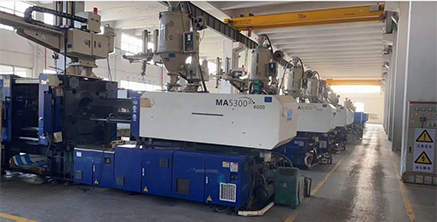+86 198 2019 2009（WhatsApp、WeChat）
Basic mechanics of injection molding molds
» » » Basic mechanics of injection molding molds

# Basic mechanics of injection molding molds

Views: 1     Author: Site Editor     Publish Time: 2022-09-23      Origin: SiteBasic mechanics of injection molding molds

The injection molding mold is to inject molten liquid plastic into the mold, and take it out after cooling and hardening.

In order to inject molten plastic, it must be pressurized.

With hydrodynamic calculations, it is possible to roughly predict how much pressure will be required.

In reality, molten plastic is viscous (sticky), and viscosity changes over time (as it cools, the viscosity increases and becomes difficult to flow), so the calculations of fluid mechanics are quite difficult formulas.

In this issue, I will explain to you the most basic "Bernoulli's theorem" when examining the state of general fluids.

Bernoulli's theorem is an equation that expresses the law of conservation of fluid energy.

【Bernoulli's Theorem】

v2/2g+p/γ+h=H

v: fluid velocity (m/sec)

g: Gravitational acceleration (9.8m/sec2)

p: fluid pressure (kgf/m2)

γ: [gamma] specific gravity of fluid (kgf/m3)The v2/2g term is called velocity head, and the p/γ term is called pressure head.

In other words, in a fluid, the energy due to velocity, the energy due to pressure, and the potential energy together determine the amount of total energy.

The Bernoulli equation is difficult to apply directly when the viscosity, fluid compressibility, and fluid velocity change, so in actual injection molding, it cannot be directly applied. However, this is an important basic formula when thinking about principles.